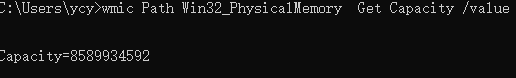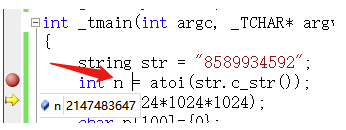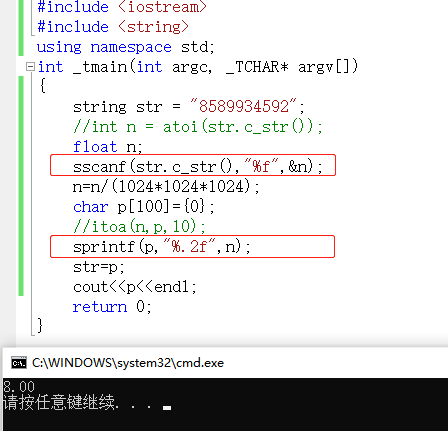• QString与stringint的相互转换: 1、QString与int的相互转换 QString qstr = QString::number(123); int i = atoi(qstr.toStdString().c_str()); 也可以这样：int i = atoi(qstr.ascii()); 2、QString与string...
QString与string、int的相互转换:

1、QString与int的相互转换
QString qstr = QString::number(123);
int i = atoi(qstr.toStdString().c_str());
也可以这样：int i = atoi(qstr.ascii());

2、QString与string（std::string）的相互转换
string s = qstr.toStdString();
QString qstr2 = QString::fromStdString(s);

展开全文• 需求：如上图为已经安装内存为8589934592Byte，字符串格式为string，需将其转换为GB显示给用户，格式依然为string； 问题： ...int n = atoi(str.c_str()); n=n/(1024*1024*1024); char p...需求：如上图为已经安装内存为8589934592Byte，字符串格式为string，需将其转换为GB显示给用户，格式依然为string；

问题：

//前部代码省略，通过命令行获取到str，这里直接将结果赋值给str
string str = "8589934592";
int n = atoi(str.c_str());
n=n/(1024*1024*1024);
char p={0};
itoa(n,p,10);
str=p;
cout<<p<<endl;//输出结果为1，为什么？


分析:

通过单步调试，发现int越界，atoi处已经越界，int范围“-2147483648~2147483647”（32位），可看出最大2开头十位数，然而实际已经8开头十位数了，然而编译器并没有报错，直接给最大数，运算后为1解决办法：

1、变量定义成float类型；

2、string转int用sscanf；

3、int转string用sprintf；TIP：1、int超过十位数建议预判越界问题；

2、sprintf和sscanf在Ｃ++功能很强大，在某些情况下远远超过atoi等这类。


展开全文• //头文件 #inculde //代码 QString a; int b; string c; b=a.toInt();//转换为int
//头文件
#inculde <QString>
//代码
QString a;
int b;
string c;
b=a.toInt();//转换为int类


展开全文• qt中将int、long型转换成char、string   char转换成QString  QString buf=QString(QLatin1String(char));//char 转换为QString QString 赋值为char  str.toStdString().c_str()   例如char tockenID;...
qt中将int、long型转换成char、string

char转换成QString
QString buf=QString(QLatin1String(char));//char 转换为QString
QString 赋值为char
str.toStdString().c_str()

例如char tockenID;
QString str=ui->lineEdit_getTokenID->text();

strcpy(tockenID,str.toStdString().c_str());

QString string int double char* 等类型的相互转化
下面总结下我知道的几种类型互转的方式：

1 QString --> string

QString.toStdString();

2 string --> QString

QString::fromStdString(string)

3 QString --->int,double,char *

QString::toInt()

QString::toDouble()

QString.toStdString().c_str();

4 int double char* --->string

可以采用<sstream>里的stringstream

以int 为例，int a = 3;

stringstream ss;

string strInt;

ss<<a;

ss>>strInt;

其他两个一样。

5 int double char*装QString

一种方法可以先转string，再转QString。另一种方法可以查看QString类的函数。

QString::number()这个静态函数，参数可以是int，也可以是double。

6 double int的互转

可采用static_cast

QString QString::number ( long n, int base = 10 ) [静态]

一个把数字n转换为字符串的方便函数，n被基于base表示，默认为10，并且必须在2到36之间。

long a = 63;

QString str = QString::number( a, 16 );         // str == "3f"

QString str = QString::number( a, 16 ).upper();   // str == "3F"

也可以参考setNum()。

实例：action/application.cpp、application/application.cpp、chart/chartform.cpp、

fonts/simple-qfont-demo/viewer.cpp、mdi/application.cpp和sql/overview/extract/main.cpp。

QString QString::number ( ulong n, int base = 10 ) [静态]

这是一个重载成员函数，提供了方便。它的行为基本上和上面的函数相同。

也可以参考setNum()。

QString QString::number ( int n, int base = 10 ) [静态]

这是一个重载成员函数，提供了方便。它的行为基本上和上面的函数相同。

也可以参考setNum()。

QString QString::number ( uint n, int base = 10 ) [静态]

这是一个重载成员函数，提供了方便。它的行为基本上和上面的函数相同。

一个把数字n转换为字符串表示的方便制造函数，n被基于base表示，默认为10，并且必须在2到36之间。

也可以参考setNum()。

展开全文• QT 关于int、double、string、QString格式相互转换...//int转double int a = 1234; double b; b = a;//直接赋值就可以 //double转int double c = 123.456; int d; d = c;//d的结果为123，即只取整数部份 d...格式转换 QString double
• //Qstring转int提供默认值，QT没有此功能函数，需要实现，qt的toInt()默认值为0 inline int ToIntDef(QString string, int defaults) { bool ok = false; int iValue = string.toInt(&ok); if(!ok) { ...c++
• ## Qtint转QString

万次阅读 多人点赞 2019-07-15 21:19:53
Qt开发,整数字符串,方法如下: int i = 5; QString s = QString::number(i);
• 我经常搞错这个问题，一直以为整形int b可以直接使用函数toString呢！ 但是在qtCreator中在整形后面不管怎么按点（可以自动提示）他就是不给...而对于int转string型直接使用toString函数即可 转载于:https://www....
• Qt 各种数据类型转换是本文介绍的内容，对于类型不同的转换，本人认为还是很好实现的。先来看内容。 AD：2013云计算架构师峰会课程资料下载 ...本文介绍的是Qt 各种数据类型转换，病...1、QString --> string QStr
• Qt5.9Creator4.4.1编译环境中，int变量QString变量的一个方法是用QString::number函数来转换，具体的用法如下所示： 1.1QString::number示例用法 quint32 MAC = 255; QString Mac1 = QString::number...QString
• 浅谈C/C++里的类型转换（char*、stringint之间相互转化）
• QString QString::number ( long n, int base = 10 ) [静态] 一个把数字n转换为字符串的方便函数，n被基于base表示，默认为10，并且必须在2到36之间。  long a = 63;  QString str = QString::number( a, 16 ...
• 自：http://blog.163.com/m_cu/blog/static/2835157820084611151913/<br />  QString QString::number ( long n, int base = 10 ) [静态] 一个把数字n转换为字符串的方便函数，n被基于base表示...
• QString QString::number ( long n, int base = 10 ) [静态]一个把数字n转换为字符串的方便函数，n被基于base表示，默认为10，并且必须在2到36之间。 long a = 63; QString str = QString::number( a, 16 ); // ...
• QString QString::number ( long n, int base = 10 ) 如: long a = 63; QString s = QString::number(a, 10); // s == "63" QString t = QString::number(a, 16)
• ## Qt中int转换成QString

千次阅读 2013-03-11 14:54:39
Qtint转换成QString  有两种方法 1.使用 QString QString::number ( long n, int base = 10 ) [static] 如: long a = 63; QString s = QString::number(a, 10); // s == "63" ...
• Qt5.12Creator编译环境中，int变量QString变量的一个方法是用QString::number函数来转换，具体的用法如下所示： 1.1QString::number示例用法 quint32MAC=255; QStringMac1=QString::number(MAC,10);//第一个...
• int  QString int m=1; QString b; b=QString::number(m) ... 转int QString a="1111" int b; b=a.toInt（） char 转换为 QString char a='b'; QString str; str=QString(a); QString 转换为
• Qtint转换成QString 有两种法使用 QString QString::number ( long n, int base = 10 ) [static] 如: long a = 63; QString s = QString::number(a, 10); // s == "63" QString
• 原博文链接在我的官方网站，网址是：http://www.aobosir.com/blog/2017/02/07/cpp-string-fusion-and-int-to-string/字符串融合#include <string>std::string str = std::string("../pairAlginClass/capture0001") +...c语言 C++ C++11
• int QString int m=1; QString b; b=QString::number(m) 2 QString 转int QString a=”1111” int b; b=a.toInt（） 3 char 转换为 QString char a=’b’; QString str; str=QString(a); 4 ...QString...